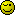# If statement not firing

Hi,

I am having problems with the code below, i donn’t get any errors, but it just does not go into the IF statement and hence does not do the INSERT statement.

So i try checking to see where the code breaks:

``````
public function insertAuthor()
{
\$authArray = array_map('mysql_real_escape_string',
\$_POST['author']);

\$query = sprintf('SELECT Pid, Pname FROM People
WHERE Pname = IN(\\'%s\\')', implode('\\',\\'', \$authArray));
\$result = mysql_query(\$query);

if(\$result && mysql_num_rows(\$result) > 0)
{
die('here');

\$PCorder = 0;
\$sqlValues = array();
while(list(\$PId, \$PName) = mysql_fetch_row(\$result))
{
if(in_array(\$PName, \$authArray))
\$sqlValues[] = sprintf("(%d, %d, now(), 0)",
\$PId, \$PCorder++ );
}

\$sql  = "INSERT INTO PeopleCon(Pid, PCorder,
";
\$sql .= implode(",\
", \$sqlValues);

die ("Generated SQL Query:<pre>\$sql</pre>");
\$result = mysql_query(\$sql);
}
}

``````

See die(‘here’);

Basically the code does not reach the IF statement, it does not die, so that means it does not like this line:

``````
if(\$result && mysql_num_rows(\$result) > 0)...

``````

I tried echoing out the \$query to see what it was showing and it displays like this:

``````
SELECT Pid, Pname FROM People
WHERE
Pname = IN('Testing 1','Testing 2')

``````

So this also seems fine, that means the IF statement is not firing.

Any ideas?

Thanks

Let me stop you right there and refer you to this page http://en.wikipedia.org/wiki/Database_normalization#Free_the_database_of_modification_anomalies

ThanksIn relation to the second insert that i want to incorporate, i think the code i posted is very wrong. The logic is to check to see if an author exists if so add in one table, if not add in both tables.

I don’t think i need to do it in the ELSE part of the if statement. Because i need to check for EVERY user in the array…

So i currently have this:

``````
public function insertAuthor()
{
\$authArray = array_map('mysql_real_escape_string',
\$_POST['author']);

\$query = sprintf('SELECT Pid, Pname FROM People
WHERE Pname IN(%s)', implode('\\',\\'', \$authArray));
\$result = mysql_query(\$query);

\$PCorder = 0;
\$sqlValues = array();

if(\$result && mysql_num_rows(\$result) > 0)
{
while(list(\$PId, \$PName) = mysql_fetch_row(\$result))
{
if(in_array(\$PName, \$authArray))
\$sqlValues[] = sprintf("(%d, %d, now(), 0)",
\$PId, \$PCorder++ );
}

\$sql  = "INSERT INTO PeopleCon(Person_id, PCorder,
";
\$sql .= implode(",\
", \$sqlValues);

\$result = mysql_query(\$sql);
}

\$p_sql  = "INSERT INTO People(Pname) VALUES ...??";
}

``````

See this line, all i need to do is INSERT into People if \$_POST[‘author’] does not already exist.

I am trying to do it here:

``````
\$p_sql  = "INSERT INTO People(Pname) VALUES ...??";

``````

But i don’t wuite know how to do this, and check for every author in the array…Hey,

Well this is actually just part of the query, just finished writing the second part, but i know theres bugs in it.

This is the full query,

``````
public function insertAuthor()
{
\$authArray = array_map('mysql_real_escape_string',
\$_POST['author']);

\$query = sprintf('SELECT Pid, Pname FROM People
WHERE Pname IN(\\'%s\\')', implode('\\',\\'', \$authArray));
\$result = mysql_query(\$query);

\$PCorder = 0;
\$sqlValues = array();

if(\$result && mysql_num_rows(\$result) > 0)
{
while(list(\$PId, \$PName) = mysql_fetch_row(\$result))
{
if(in_array(\$PName, \$authArray))
\$sqlValues[] = sprintf("(%d, %d, now(), 0)",
\$PId , \$PCorder++ );
}

\$sql  = "INSERT INTO PeopleCon(Person_id, PCorder,
";
\$sql .= implode(",\
", \$sqlValues);

\$result = mysql_query(\$sql);
} else {
while(list(\$PId, \$PName) = mysql_fetch_row(\$result))
{
if(in_array(\$PName, \$authArray))
\$sqlValues[] = sprintf("(%d, %d, now(), 0)",
\$PId, \$PCorder++ );
\$peopleValues[] = sprintf("(%d, now(), 0)",
\$_POST['author']);
}

\$sql  = "INSERT INTO PeopleCon(Person_id, PCorder,
";
\$sql .= implode(",\
", \$sqlValues);
\$result = mysql_query(\$sql);

\$p_sql  = "INSERT INTO People(Pname, Pdateadded,
Pdeleted) VALUES \
";
\$p_sql .= implode(",\
", \$peopleValues);
\$p_result = mysql_query(\$p_sql);
}
}

``````

Firstly, i have 2 of the same INSERT statement in both sets of if conditions, which i am sure this can be prevented somehow? Also what this function should be doing is firstly checking to see if an author exists, if so, INSERT them into ONLY the PeopleCon table, which works.

If not, then INSERT them into both People AND PeopleCon…

Where am i going wrong?

Since you already santize data here

You don’t need the single quotes hereThanks!Been sat at a laptop for too longEven better would beRemove the = from your query – that’s not valid syntax.

``````SELECT
Pid
, Pname
FROM
People
WHERE
Pname
IN
(
'Testing 1'
, 'Testing 2'
)

``````